##### Lecture 1 Newton's 1st and 2nd Laws

0
Set Details Share
created 8 years ago by Annabelle
36 views
updated 8 years ago by Annabelle
College: Second year
Subjects:
physics
Page to share:
Embed this setcancel
COPY
code changes based on your size selection
Size:
X
1

t

time
seconds [s]

2

M

mass
kilograms [kg]

3

f

force
newtons [N]

4

X

position
meters [m]

5

ΔX

change in postion

6

V

velocity [m/s]

7

a

acceleration [m/s2]

8

What happens when a tennis ball is released from you hand?

Gravity causes the ball to fall
Velocity increases
change in position
change in velocity

9

Velocity

change in position/time
Speed with direction
60 mph vs. 60 mph north

10

acceleration

change in velocity/time

11

Force

push or pull

12

Mass

measure of the amount of stuff
resistance to change in motion

13

Newton’ second Law

acceleration is proportional to force and inversely proportional to mass
a = F/M or F = MA

14

Net Force

sum of forces
ΣF=ma

15

When F = 0 then a = 0

equilibrium
static of dynamic

16

static equilibrium

at rest

17

dynamic equilibrium

moving at constant velocity

18

Newton’s 1st law

Law of Inertia
an object at rest will stay at rest and an object in motion will move with constant velocity unless acted upon by an external force

19

Friction

force that opposes motion

20

Inertia

tendency for an object to maintain motion

21

Force of Gravity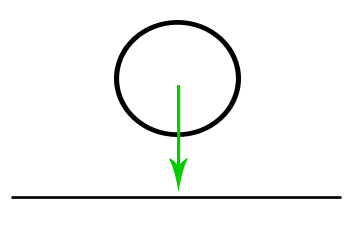weight
“free fall”
If gravity is the only force acting on an object then the object, will free fall. Force of gravity always straight down

22

Drag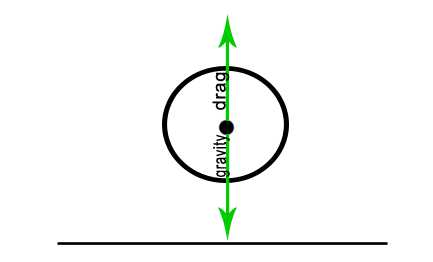force upward

23

Terminal Velocity

When drag = weight constant speed results (ex. parachuting)

dynamic equilibrium

24

Weight always straight down but normal force not always straight up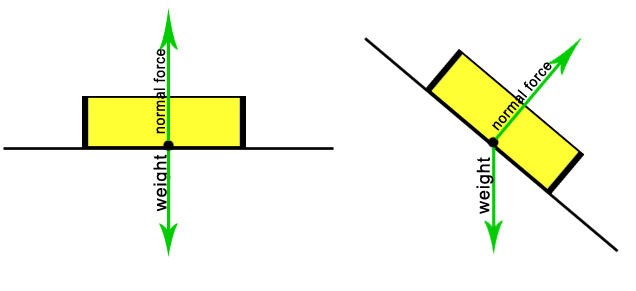25

Tension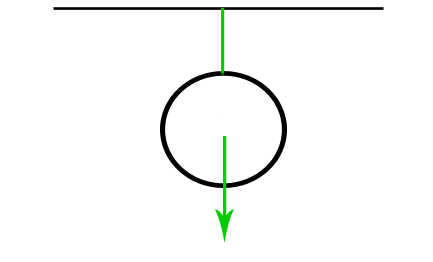pulling force exerted by a string, cable, chain, or similar solid

26

Aerodynamic forces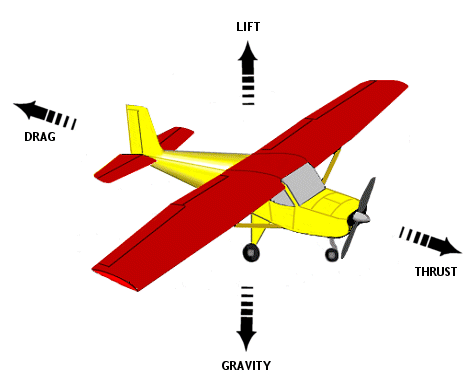27

F = ma

force = mass/acceleration
[N] = [kg][m/s2]

28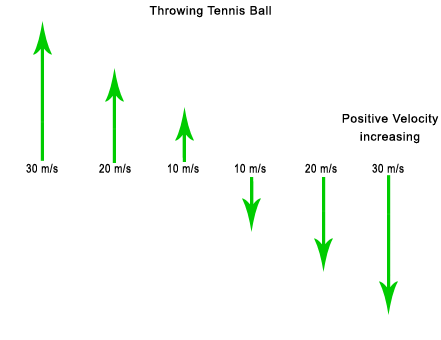...

29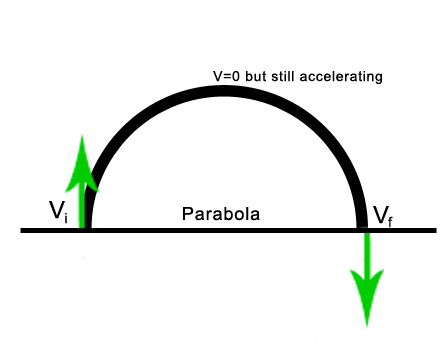...

30

Maximum Height

ΔX = Vt when a = 0

ΔX = Vaverage t